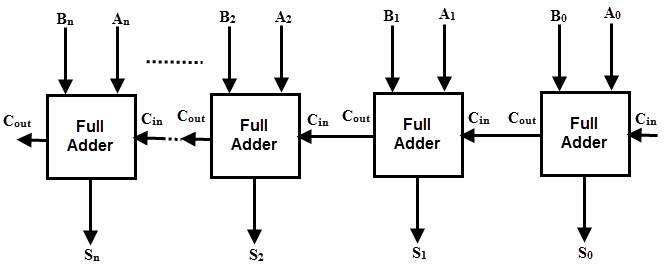#Difference Between Similar Terms and Objects

Full adder and half adder are digital circuit elements used to summarize numbers. In modern computers they are part of the arithmetic logical unit that is responsible for carrying out arithmetic operations. Adders work with electrical signals representing the binary numbers of computers. In electronics, the adder is a digital circuit that is adding numbers. In many computer and other processors, adders are not only used in the arithmetic-logical unit, but also in other parts where it is necessary to calculate the address, index tables and similar operations. Although adders can be constructed for various representations of numbers, such as binary coded decimal numbers, they are usually binary-numbered bitters.The truth table used to describe the work of a half adder is:

 a b S C 0 0 0 0 0 1 1 0 1 0 1 0 1 1 0 1

where S=a⊕b; C=a*b

The truth table used to describe the work of a full adder is:

 a b Cin S Cou 0 0 0 0 0 0 0 1 1 0 0 1 0 1 0 0 1 1 0 1 1 0 0 1 0 1 0 1 0 1 1 1 0 0 1 1 1 1 1 1

where S=a⊕b⊕Cin; Cout=(a*b)+(Cin*(a⊕b))1. ### Definition of Half  and Full Adder

Both half adder and full adder are combinational circuits. However, the first one adds two one bit digits, while the second one adds three digits.

1. ### Input of Half  and Full Adder

The half adder has two input values – a and b which represent the data bits. The full adder has additional input – the carry from the lower class (Cin).

1. ### Output of Half  and Full Adder

The half adder has two outputs. One is the sum of the process (S) and the other is the carry of the summation (C). The full adder also has two outputs (S;Cout).

1. ### Carry

In case of a half adder the carry from the lower class (previous iteration) is not added in the new class. In case of full adder the carry is transferred in the new class, which allows the adder to sum up numbers.

1. ### Components of Half  and Full Adder

The half adder consists of XOR and an AND gate. The full adder is principally two half adders combined together – consists of two XOR and two AND gates and an OR gate.

1. ### Usage of Half  and Full Adder

Half adders are used in computers, calculators, measuring devices etc. Full adders are used in digital processing.## Summary of Half  Verses Full Adder

• When two binary numbers are added, firstly the digits are added, i.e. the smallest bits. This operation is performed by a half adder as the simplest network that allows to sum up two one-bit numbers. The input signals in the network are the binary digits of the digits, and the resulting outputs are the sum and the carry.
• When multi-digit numbers are added, the half adder network can be used only to assemble the smallest digits, since the half adder cannot sum up the carry digit from the previous class. The basis of all digital arithmetic devices is the so-called full adder. This network is used to add three single digit numbers. Two numbers are assigned, and the third is the carry from the previous class calculations.

### Search DifferenceBetween.net :

Custom Search

Help us improve. Rate this post!Loading...Email This Post : If you like this article or our site. Please spread the word. Share it with your friends/family.

### 1 Comment

1. Useful information. Thank you

Please note: comment moderation is enabled and may delay your comment. There is no need to resubmit your comment.

## References :

Givone D. D., "Digital Principles and Design", NY: McGraw-Hill, 2002

Leach, D. P., Malvino, A. P. “Experimental in Digital Principles”, 5th Ed., NY: McGraw-Hill, 1994

Bartee, T.C. “Digital Computer Fundamentals“, 6th Ed., NY: McGraw-Hill, 1985